407 493 6601

# Algebra 1 - Linear Equations

## Introduction

• Linear equations are equations of degree one. For example, $3x-5y=17$ is a linear equation in two variables.
• The equation of a straight line is also a linear equation.

## Slope or Gradient of a line

• The slope of a line is the ratio of change in the y-coordinates to the change in the x-coordinates.
• The slope $\left(m\right)$ of a non-vertical line passing through the points $\left({x}_{1},{y}_{1}\right)$ and $\left({x}_{2},{y}_{2}\right)$ is given by: $m=\frac{{y}_{2}-{y}_{1}}{{x}_{2}-{x}_{1}},$${x}_{1}\ne {x}_{2}$.
• The formula for slope is also referred to as rise over run.
• The slope of a line parallel to the x-axis is zero.
• The slope of the y-axis is not defined.
• If two lines are parallel, their slopes are equal.
• If two lines are perpendicular to each other, the product of their slopes is -1.

## Forms of Linear Equation

• The standard form of a line: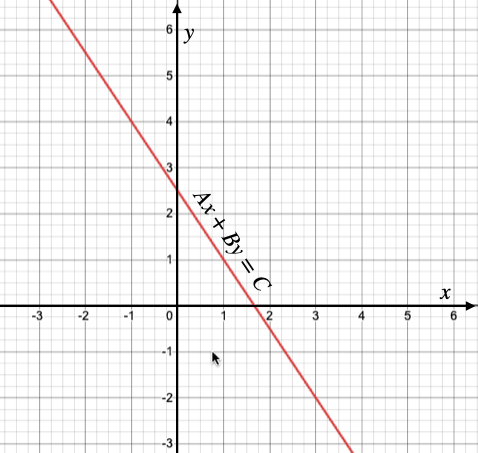• In the standard form, a linear equation is expressed as: $Ax+By=C$, where $A,B\ne 0$.
• The Slope-Intercept form of a line: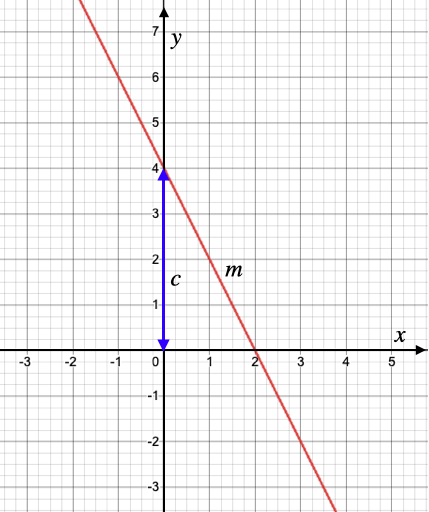•  The equation of a line with slope $m$, and making an intercept $c$ on y-axis is given by $y=mx+c$.
• The Point Slope form of a line: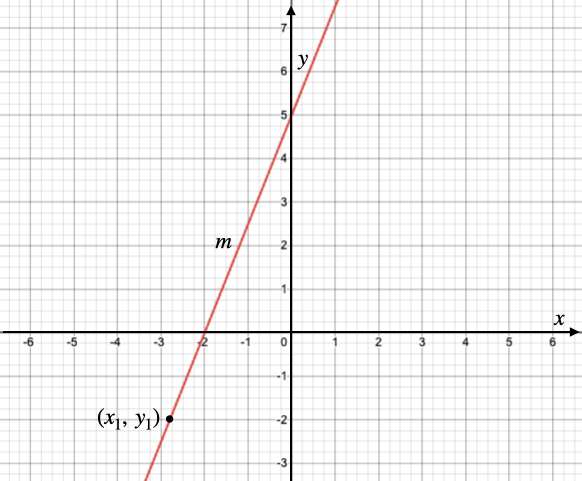• The equation of a line with slope $m$, and passing through a point $\left({x}_{1},{y}_{1}\right)$ is given by $y-{y}_{1}=m\left(x-{x}_{1}\right)$.
• The Two Point form of a line: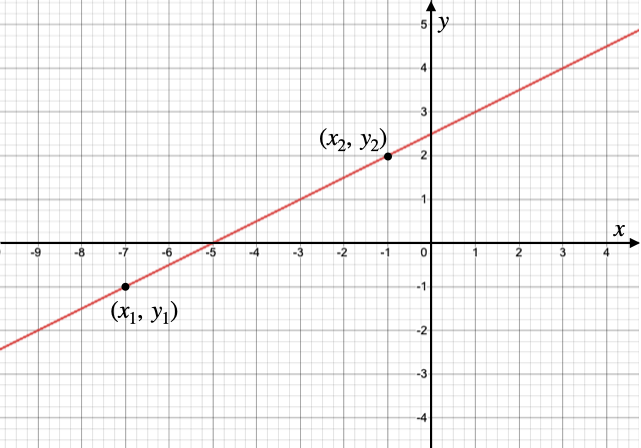• The equation of a line passing through the points $\left({x}_{1},{y}_{1}\right)$ and $\left({x}_{2},{y}_{2}\right)$ is $\left(y-{y}_{1}\right)=\frac{{y}_{2}-{y}_{1}}{{x}_{2}-{x}_{1}}\left(x-{x}_{1}\right)$.
• Equation of a line in Intercept form: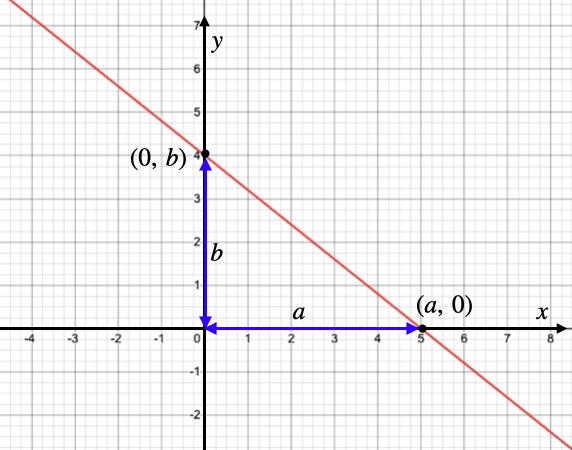• The equation of a line that makes intercepts of lengths $a$ and $b$ on the x-axis and y-axis respectively is given by $\frac{x}{a}+\frac{y}{b}=1$.

## Solved Examples

Question 1: Find the slope of a line passing through the points (-3, 5) and (7, 4).

Solution: Here, ,

Slope, $m=\frac{{y}_{2}-{y}_{1}}{{x}_{2}-{x}_{1}}$$=\frac{4-5}{7-\left(-3\right)}$$=\frac{-1}{7+3}$$=-\frac{1}{10}$

Question 2: Find the equation of a line with slope, $m=\frac{2}{5}$ and passing through the point $\left(-2,1\right)$.

Solution: The point-slope form of a line is given by the equation $\left(y-{y}_{1}\right)=m\left(x-{x}_{1}\right)$

Here, , and $m=\frac{2}{5}$.

So, the desired equation will be $\left(y-1\right)=\frac{2}{5}\left[x-\left(-2\right)\right]$

$5\left(y-1\right)=2\left(x+2\right)$

$5y-5=2x+4$

$2x-5y+9=0$

Question 3: Find the equation of a line passing through the points (1, -3) and (2, 5).

Solution: Here, .

slope $m=\frac{\left({y}_{2}-{y}_{1}\right)}{\left({x}_{2}-{x}_{1}\right)}$$=\frac{5-\left(-3\right)}{2-1}$$=\frac{5+3}{1}$$=8$

The equation of the line will be $\left(y-{y}_{1}\right)=m\left(x-{x}_{1}\right)$

$\left[y-\left(-3\right)\right]=8\left(x-1\right)$

$\left(y+3\right)=8x-8$

$y+3=8x-8$

$8x-y=11$

## Cheat Sheet

• Linear equations are equations in which the highest power (degree) of the variable is 1.
• The slope $\left(m\right)$ of a non-vertical line passing through the points $\left({x}_{1},{y}_{1}\right)$, and $\left({x}_{2},{y}_{2}\right)$ is given by $m=\frac{{y}_{2}-{y}_{1}}{{x}_{2}-{x}_{1}},$${x}_{1}\ne {x}_{2}$.
• Equation of a line in standard form: $Ax+By=C$, where $A$ and $B$ are non-zero coefficients of x and y, respectively.
• The Slope-Intercept form of a line: The equation of a line with slope $m$, and making an intercept $c$ on y-axis is given by $y=mx+c$.
• The Point Slope form of a line: The equation of a line with slope $m$, and passing through a point $\left({x}_{1},{y}_{1}\right)$ is given by $y-{y}_{1}=m\left(x-{x}_{1}\right)$.
• The Two Point form of a line: The equation of a line passing through the points $\left({x}_{1},{y}_{1}\right)$ and $\left({x}_{2},{y}_{2}\right)$ is $y-{y}_{1}=\frac{{y}_{2}-{y}_{1}}{{x}_{2}-{x}_{1}}\left(x-{x}_{1}\right)$.
• Equation of a line in Intercept form: The equation of a line that makes intercepts of lengths $a$ and $b$ on the x-axis and y-axis respectively is given by $\frac{x}{a}+\frac{y}{b}=1$.
• Two lines are parallel if and only if their slopes are equal.
• Two lines are perpendicular if and only if the product of their slopes is -1.

## Blunder Areas

• Remember that the slope of a vertical line is not defined.
• In the slope-intercept form of a line, $y=mx+c$$c$ is the y-intercept and must not be confused with the x-intercept.